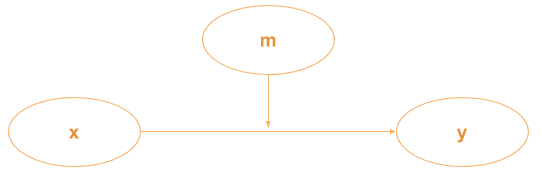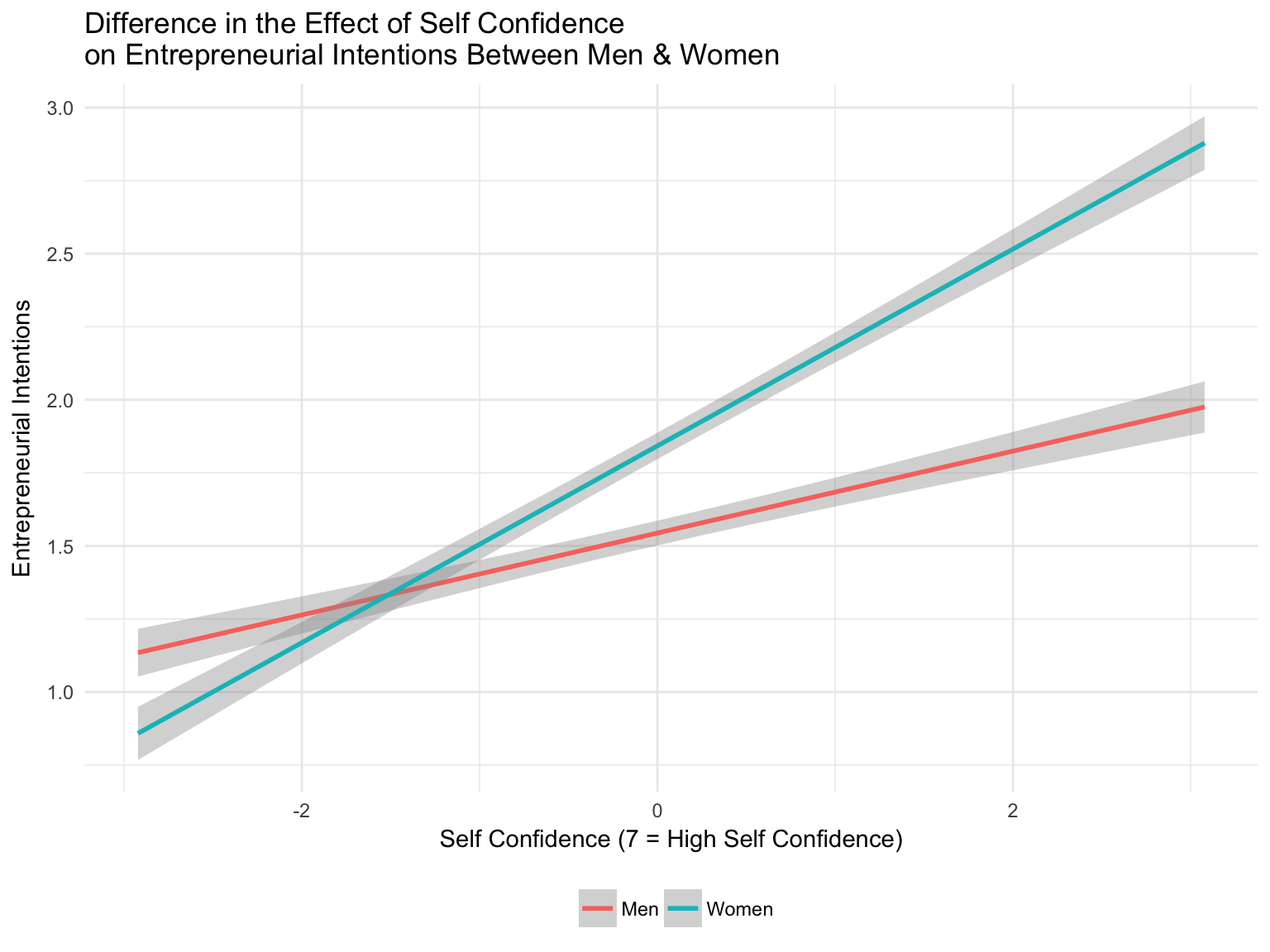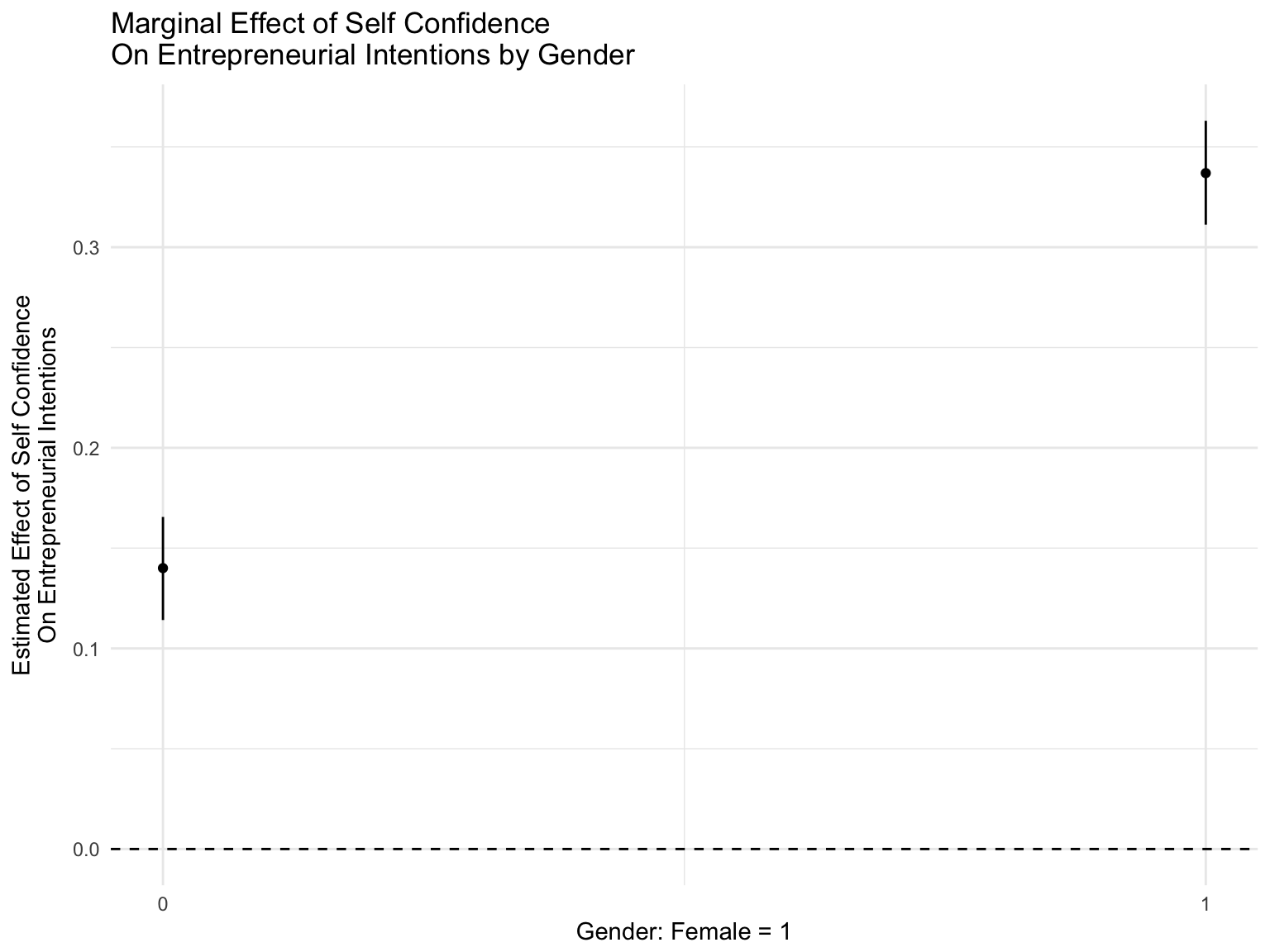Moderation: The Basics

ENT5587B - Research Design & Theory Testing II

Brian S. Anderson, Ph.D.
Assistant Professor
Department of Global Entrepreneurship & Innovation
andersonbri@umkc.edu© 2017 Brian S. Anderson

• How goes it?
• IRB Applications
• Paper progress
• The basics of moderation
• Group comparisons
• Confidence intervals and simple slopes
• Marginal effects
• Lab 9 Feb: Moderation Assessment
$$y=\alpha+{\beta}{x}+{\beta}{m}+{\beta}{x}{m}+\varepsilon$$These are the same thing. I prefer the equation format, some prefer the graphical format. Nonetheless, we interpret them the same way.

In moderation, we’re saying that the effect of x on y varies as a function of the level of m.

$$y=\alpha+{\beta}{x}+{\beta}{m}+{\beta}{x}{m}+\varepsilon$$

Ok, that definition is accurate, but not very helpful.

We’re going to explore moderation in depth over the next few weeks, but I think it’s helpful to think about moderators in three different ways (although the equation/model is the same)…

The moderator is a boundary condition.

For example, the relationship between x an y only manifests in the presence of m.

The moderator changes the nature of the relationship between x and y.

For example, at one level of the moderator, the relationship between x and y is positive; at another level, the relationship between x and y is negative.

The moderator changes the strength, or degree of the relationship between x and y.

For example, the positive relationship between x and y is more positive in the presence of a particular level of m.

Before moving on, I can’t stress this point enough…

The type of moderation effect MUST be derived from theory.

The hunt for astericks is especially pernicious when it comes to moderation effects in our literature. So much so that I would place the conditional probability of false/misleading moderation effects at greater than 75%.

Yes, I think it’s that bad, and I’m not the only one.

The reasons vary, as you read about. The material point though is that while interaction effects are theoretically interesting and practically useful, it is easy to use and abuse frequentist statistics to find moderation effect coefficients with p < .05.

It is also common to have reviewers suggest post hoc moderation hypotheses, which is also decidedly unhelpful.

Oh, endogeneity is also a significant problem in moderation models, particularly regarding selection effects, measurement error, omitted variables, etc.

To be clear, I’m in the “mend it” camp.

But, we’ve got a long way to go as a field, because old habits die hard.

I think the best way to understand moderation is by running a model. We’re going to start with a dichotomous moderator.

While not used as often as it could (should) be, dichotomous moderators generally represent group membership. The group could be industry membership, hair color, whether you are a Broncos fan or not, or in our case today, gender.

So to get started, lets create a dataset to use (yes, we will be doing this a lot). We’re going to need a continuous y, a continuous x, and a dichotomous moderator, m.

But unlike last week, we really want to show a moderation effect. So instead of just generating a random dataset, we’re going to actually simulate data based on a regression equation that we’ve determined in advance.

So lets set up our theoretical model. We want to predict the effect of self-confidence on entrepreneurial intentions. Specifically, we believe that self-confidence has a strong (i.e., effect size greater than .3) positive effect on desire to be an entrepreneur.

But we also believe that gender changes the degree of this relationship, such that among women, the effect of self-confidence on entrepreneurial intentions is stronger (i.e., has a steeper slope) than among men.

We’re going to measure self-confidence with a single indicator, seven-point Likert scale with 7 being very self-confident. Gender is a dichotomous variable with 1 equal to female.

Lets walk through the logic for our equation…

$$y=\alpha+{\beta}{x}+{\beta}{m}+{\beta}{x}{m}+\varepsilon$$

Now lets modify that equation based on the names of our variables:

$$EntIntentions=\alpha+{\beta}{SelfConfidence}+{\beta}{Gender} +...$$ $${...\beta}{SelfConfidence*}{Gender}+\varepsilon$$

A question often comes up in moderation about why we multiply the two independent variables together to investigate the interaction effect.

It’s easier to see why with a dichotomous moderator.

Take our equation, but this time, set Gender = 0; this is the effect of self-confidence on entrepreneurial intentions among men:

 $$EntIntentions=\alpha+{\beta}{SelfConfidence}+{\beta}{Gender} +...$$ $${...\beta}{SelfConfidence*}{Gender}+\varepsilon$$

 $$EntIntentions=\alpha+{\beta}{SelfConfidence}+{\beta}{Gender*0} +...$$ $${...\beta}{SelfConfidence*}{Gender*0}+\varepsilon$$

 $$EntIntentions=\alpha+{\beta}{SelfConfidence}+\varepsilon$$

Our equation reduces to just the simple slope of self-confidence on entrepreneurial intentions for this group. This is, effectively, our baseline (or reference) effect.

Now lets look at the equation with Gender = 1, which is our effect among women:

 $$EntIntentions=\alpha+{\beta}{SelfConfidence}+{\beta}{Gender} +...$$ $${...\beta}{SelfConfidence*}{Gender}+\varepsilon$$

 $$EntIntentions=\alpha+{\beta}{SelfConfidence}+{\beta}{Gender*1} +...$$ $${...\beta}{SelfConfidence*}{Gender*1}+\varepsilon$$

In both regression models (Gender = 0 and Gender = 1), the equation will estimate the effect of Gender on Entrepreneurial Intentions. But when we get the predicted values of that equation for the Gender = 0 condition, we multiply the Gender coefficient by zero, and the interaction coefficient by zero, which gives us the baseline effect (slope).

But in the Gender = 1 condition, we’re going to add that effect back in to our predicted values, and add the term for the interaction effect into the prediction.

Effectively, we’re creating a partialed effect of the the relationship between Self-Confidence and Entrepreneurial Intentions in the Gender = 1 condition, while holding constant the mean effect of Self-Confidence (which in our case, is zero).

So, assuming a significant interaction effect consistent with our hypothesis, we should see the slope of the relationship between Self Confidence and Entrepreneurial Intentions get steeper for women as opposed to men.

Sidebar…

There is an interesting statistical theory discussion about whether you actually need to include the lower order terms in a regression model.

I’m mixed on the issue, but convention (and some logic) says to include the lower order terms.

Now, over the coming weeks we’ll tackle the myth that mean-centering or standardizing interaction terms addresses multicollinearity (it doesn’t), and we’ll also tackle the myth that you cannot interpret the lower-order term coefficients in an interaction model (you can).

End sidebar.

Ok, lets get to the data! We’re going to start by randomly generating our x and our m. Our x will be standard normal, but will range from 1 to 7 (mimicking a Likert scale). Our m will be dichotomous (0/1).

library(tidyverse)
set.seed(08022003)
my.df <- data_frame(SelfConfidence = round(runif(1000,1,7),0),
Gender = rbinom(1000,1,.5))
# Center Self Confidence at its mean value
my.df$SelfConfidence <- scale(my.df$SelfConfidence, center = TRUE,
scale = FALSE)

Now we’re going to build our specified regression model:

$$EntIntentions=\alpha+{\beta}{SelfConfidence}+{\beta}{Gender} +...$$ $${...\beta}{SelfConfidence*}{Gender}+\varepsilon$$

We’re going to start by assigning values to our coefficients…

my.effects <- data_frame(a = 1.5,  # This is our alpha
b1 = 0.15,  # This is the effect of x on y
b2 = .35,  # This is the effect of m on y
b3 = .2)  # This is the interaction effect

One more step to building our model…

Remember a regression equation has a disturbance term, $$\varepsilon$$, that must have a constant variance for the model to render consistent estimates. So we need to generate a random set of numbers for our disturbance term, but we’re going to force that vector to have a constant standard deviation (the square root of the variance) and an expected mean of zero.

sd <- .5
mu <- 0
my.df$epsilon <- rnorm(1000, mu, sd) # Add this to our dataframe Now we’re ready to specify our model and generate our predicted values for y. my.df$EntIntentions <- my.effects$a + (my.effects$b1 * my.df$SelfConfidence) + (my.effects$b2 * my.df$Gender) + (my.effects$b3 * my.df$SelfConfidence * my.df$Gender) +
my.df$epsilon So lets see how we did (we’ll come back to the model code later)… ## # A tibble: 1 × 4 ## a b1 b2 b3 ## <dbl> <dbl> <dbl> <dbl> ## 1 1.5 0.15 0.35 0.2 summary(lm(EntIntentions ~ SelfConfidence*Gender, data = my.df)) ## ## Call: ## lm(formula = EntIntentions ~ SelfConfidence * Gender, data = my.df) ## ## Residuals: ## Min 1Q Median 3Q Max ## -1.48924 -0.33153 0.00429 0.34522 1.98068 ## ## Coefficients: ## Estimate Std. Error t value Pr(>|t|) ## (Intercept) 1.54414 0.02229 69.263 <2e-16 *** ## SelfConfidence 0.14015 0.01283 10.922 <2e-16 *** ## Gender 0.29824 0.03159 9.441 <2e-16 *** ## SelfConfidence:Gender 0.19659 0.01829 10.748 <2e-16 *** ## --- ## Signif. codes: 0 '***' 0.001 '**' 0.01 '*' 0.05 '.' 0.1 ' ' 1 ## ## Residual standard error: 0.4992 on 996 degrees of freedom ## Multiple R-squared: 0.473, Adjusted R-squared: 0.4714 ## F-statistic: 297.9 on 3 and 996 DF, p-value: < 2.2e-16 Lets get down to work now… There are a number of packages available to evaluate moderation models. We’re going to start with just the built in lm function, which was the one that we just used. # Note that the '*' operator is an R shortcut to create the interaction. # This shortcut also tells R to include the lower order terms. interaction.model <- lm(EntIntentions ~ SelfConfidence*Gender, data = my.df) summary(interaction.model) ## ## Call: ## lm(formula = EntIntentions ~ SelfConfidence * Gender, data = my.df) ## ## Residuals: ## Min 1Q Median 3Q Max ## -1.48924 -0.33153 0.00429 0.34522 1.98068 ## ## Coefficients: ## Estimate Std. Error t value Pr(>|t|) ## (Intercept) 1.54414 0.02229 69.263 <2e-16 *** ## SelfConfidence 0.14015 0.01283 10.922 <2e-16 *** ## Gender 0.29824 0.03159 9.441 <2e-16 *** ## SelfConfidence:Gender 0.19659 0.01829 10.748 <2e-16 *** ## --- ## Signif. codes: 0 '***' 0.001 '**' 0.01 '*' 0.05 '.' 0.1 ' ' 1 ## ## Residual standard error: 0.4992 on 996 degrees of freedom ## Multiple R-squared: 0.473, Adjusted R-squared: 0.4714 ## F-statistic: 297.9 on 3 and 996 DF, p-value: < 2.2e-16 So what does it mean? Well, since both Self Confidence and Gender have meaningful zero values (mean and male, respectively), we catch a bit of a break in making sense of the output. Coefficient Interpretation $${\beta}$$ SelfConfidence Average expected change in Entrepreneurial Intentions for a one unit change in Self Confidence, when Gender equals zero and the interaction effect also equals zero (the baseline) $${\beta}$$ Gender Average expected change in Entrepreneurial Intentions as Gender shifts from Male to Female, holding Self Confidence at the mean (zero) and the interaction effect at zero $${\beta}$$ SelfConfidence*Gender Average expected change in the effect of Self Confidence on Entrepreneurial Intentions Ok, truth be told, it’s hard to make sense of an interaction effect from just the coefficients. What you can say, however, is the presence of a statistically significant coefficient estimate for the interaction term provides evidence that the average effect (i.e., the slope) of self-confidence on entrepreneurial intentions is significantly different between men and women. This is what is known as the simple slope comparison. Simple slopes just tell part of the story though. While our moderator is dichotomous, our focal predictor—self-confidence—is continuous (at least we’re treating it that way). Our coefficient estimates though just speak to the average change in entrepreneurial intentions. While on average the slopes are different between men and women, this difference might not hold across all of the observed values of self-confidence. We’ll come back to this more when we get in to continuous by continuous interaction effects next week. Right now though, the average effect that we’re talking about really is just the difference in the simple slopes because we have a dichotomous moderator. While the coefficient estimate did give us statistically significant evidence that the slopes were different, a visualization is a better way to make sense of the interaction effect. Oh, ALWAYS plot confidence intervals with an interaction effect!!! library(ggthemes) # Lets set Gender to a proper factor variable my.df$Gender <- factor(my.df\$Gender, labels = c("Men", "Women"))
interaction.plot <- ggplot(data = my.df,
aes(x = SelfConfidence, y = EntIntentions,
group = Gender, colour = Gender))
interaction.plot + stat_smooth(method="lm") +
theme_minimal() +
theme(legend.position = "bottom") +
xlab("Self Confidence (Mean Centered)") +
ylab("Entrepreneurial Intentions") +
labs(colour="") +
ggtitle("Difference in the Effect of Self Confidence\non Entrepreneurial Intentions Between Men & Women")
With self confidence centered, we can see that there is a range of values where there is no difference in the slopes (effect) of self confidence on entrepreneurial intentions between men and women.There are a lot of other packages that let you construct interaction plots, including one from our friends down I-70 called rockchalk. I prefer though using ggplot for consistency and flexibility, but you can certainly experiment!

Lastly, lets talk about marginal effects. This will be more important when we get to continuous by continuous interactions, but it’s helpful to go through this now.

Similar to our discussion of LDV models and changing probabilities, interaction models share the same feature. Assuming your hypothesis is correct, the slope (effect) of your focal predictor (x) changes as a function of the presence (level) of the moderator.

The marginal effect then of an interaction model is the difference between these differences.

Because our model had a dichotomous moderator, the marginal effect in this case is literally just the average difference between the two estimated slopes. There really isn’t much to calculate here, because our statistically significant interaction effect (SelfConfidence X Gender) tells us that the slopes are different, and the coefficient estimate is the average marginal effect; the shift in the slope from our baseline condition.

But, it can be helpful to visualize marginal effects, and we’ll be using this package a lot over the coming weeks…

install.packages("interplot")
library(interplot)
interplot(m = interaction.model, var1 = "SelfConfidence", var2 = "Gender") +
geom_hline(yintercept = 0, linetype = "dashed") +
theme_minimal() +
xlab("Gender: Female = 1") +
ylab("Estimated Effect of Self Confidence\nOn Entrepreneurial Intentions") +
ggtitle("Marginal Effect of Self Confidence\nOn Entrepreneurial Intentions by Gender")Wrap-up.

Lab 9 Feb – Moderation Assessment

Seminar 13 Feb – Moderation, Part II